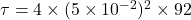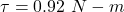## A plastic wire is wind on a wheel on a radius 3 cm. The whole system is initially at rest. A force is applied on the plastic wire and the wi

Question

A plastic wire is wind on a wheel on a radius 3 cm. The whole system is initially at rest. A force is applied on the plastic wire and the wire unwinds from the wheel from its axis of rotation. The wheel is given an angular acceleration of 92.00 rad/s2 for:______.

in progress 0
2 months 2021-07-30T06:17:43+00:00 1 Answers 1 views 0

The torque of the wheel is 0.92 N-m.

Explanation:

Given that,

Radius of wire = 3 cm

Suppose, Mass of wheel is 4 kg and radius is 5 cm. find the torque of the wheel.

We need to calculate the torque of the wheel

Using formula of torque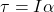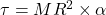Where, M = mass of wheel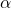= angular acceleration